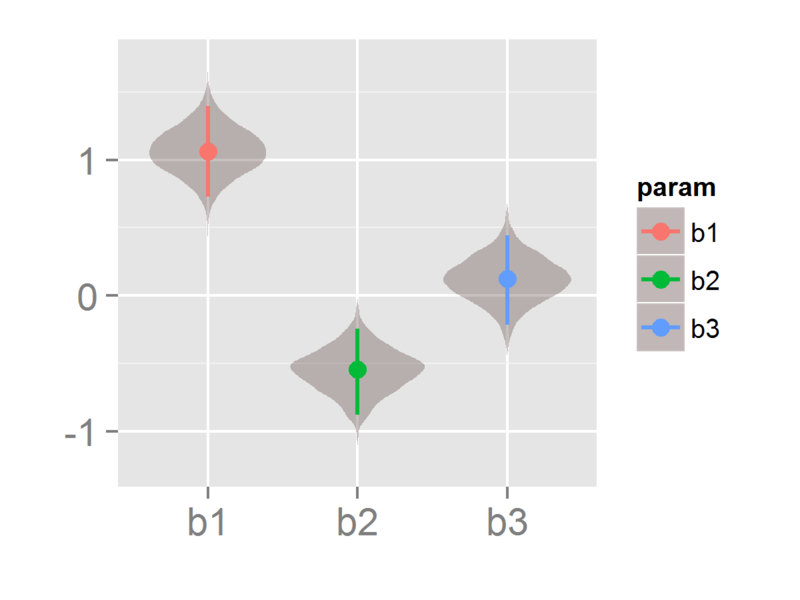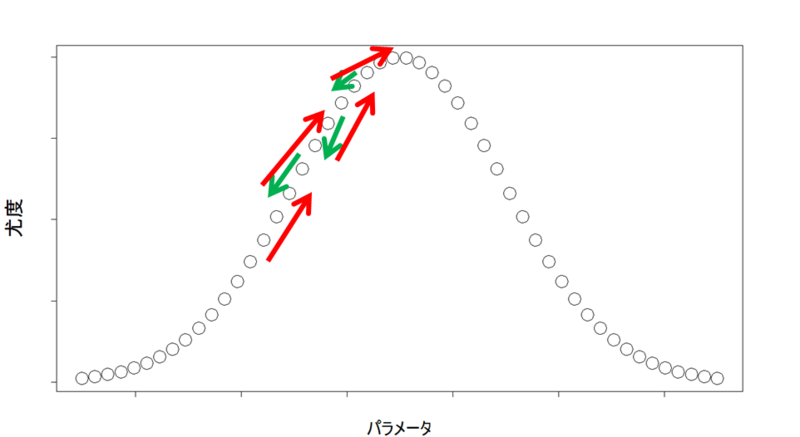# Bayesian modeling with R and Stan (1): Overview

Although I've written a series of posts titled "Machine Learning for package uses in R", usually I don't run machine learning on daily analytic works because my current coverage is so-called an ad-hoc analysis.

Instead of machine learning, ad-hoc analysts often use statistical modeling such as linear models (called "multiple regression" in general), generalized linear models (GLM) and/or econometric time series analysis. But in some situations such linear model and its variants would not work because of nonlinear components and/or individual variance, called "random effect".

In general, random effect can be well handled by generalized linear mixed models (GLMM) and for example CRAN has some related packages. But in some cases random effects cannot be formulated concisely and explicitly... if so, we have a strong alternative method to resolve it: "Bayesian using Markov Chain Monte Carlo (MCMC) method".As one of the strongest methods for ad-hoc analysis, a series of posts will argue about Bayesian modeling with MCMC and its apllication. For the first time, this post overviews it.

### Why MCMC?

As well known, generalized linear models use maximum likelihood estimation in order to estimate parameters such as coefficients or intercept. But when a likelihood function is too complicated to analytically solve -- please imagine a likelihood equation with a complicated mixture of various dimensional trend terms, seasonal term, irregular perturbation term in addition to usual multivariate linear combination -- , no numerical methods such as Newton-Raphson method can compute maximum likelihood.

One of the most efficient solution is MCMC; while its computational cost is huge, MCMC can provide a posterior probability distribution itself for likelihood and each parameter and then parameters can be easily estimated.In principle Monte Carlo sampling is merely one of numerical (in particular integral) methods, but with some improvement such as Markov chain, MCMC is widely used in maximum likelihood estimation for statistical modeling.

### Why Stan?

For years, "BUGS" (Bayesian using Gibbs Sampling) project software has been widely used, such as WinBUGS or OpenBUGS. But for some reasons currently it's not updated any longer and perhaps nobody supports it.

In addition BUGS software has a serious disadvantage: very slow. Maybe its implementation would be out-of-date (for Windows XP era!!!) so now it cannot satisfy recent system requirements.

Although there are some alternatives such as {MCMCpack} CRAN package or JAGS (Just Another Gibbs Sampler), these days Stan has been widely used.

Stan uses Hamilton Monte Carlo (HMC) and No U-Turn Sampling (NUTS) and is implemented by C++ in order to speed up computation. In turn, unlike BUGS software Stan cannot handle discrete variables because HMC requires integral computation and it cannot compute that of discrete variables while BUGS uses Gibbs Sampler that can generate MCMC samples from discrete variables.